﻿ 岛式上层建筑总强度计算方法
 舰船科学技术2019, Vol. 41Issue (8): 12-16PDF

Research on calculation method for general strength of island superstructure
YU Bin, CHEN Zhi-jian, TANG Yu-hang
Department of Naval Architecture Engineering, Naval University of Engineering, Wuhan 430033, China
Abstract: Research on the load reason and loading ways of the overall strength of the island structure. Based on the theory of finite element and the theory of hull strength, proposed a new method for calculating the strength of island structure. The method is well suited to the current situation of the hull strength. Which can effectively used the calculation results of the hull strength in the calculation process of the ship, and overcame the shortcomings of the traditional calculation method of the overall strength of the superstructure and meet the requirements of structural optimization design. The finite strip method and the finite element method were used to calculate the overall strength of the superstructure of a ship, and finally found that the results of finite strip method are smaller.
Key words: island structure     the finite strip method     the finite element method     overall strength calculation
0 引　言

1 上层建筑总强度计算理论及载荷分析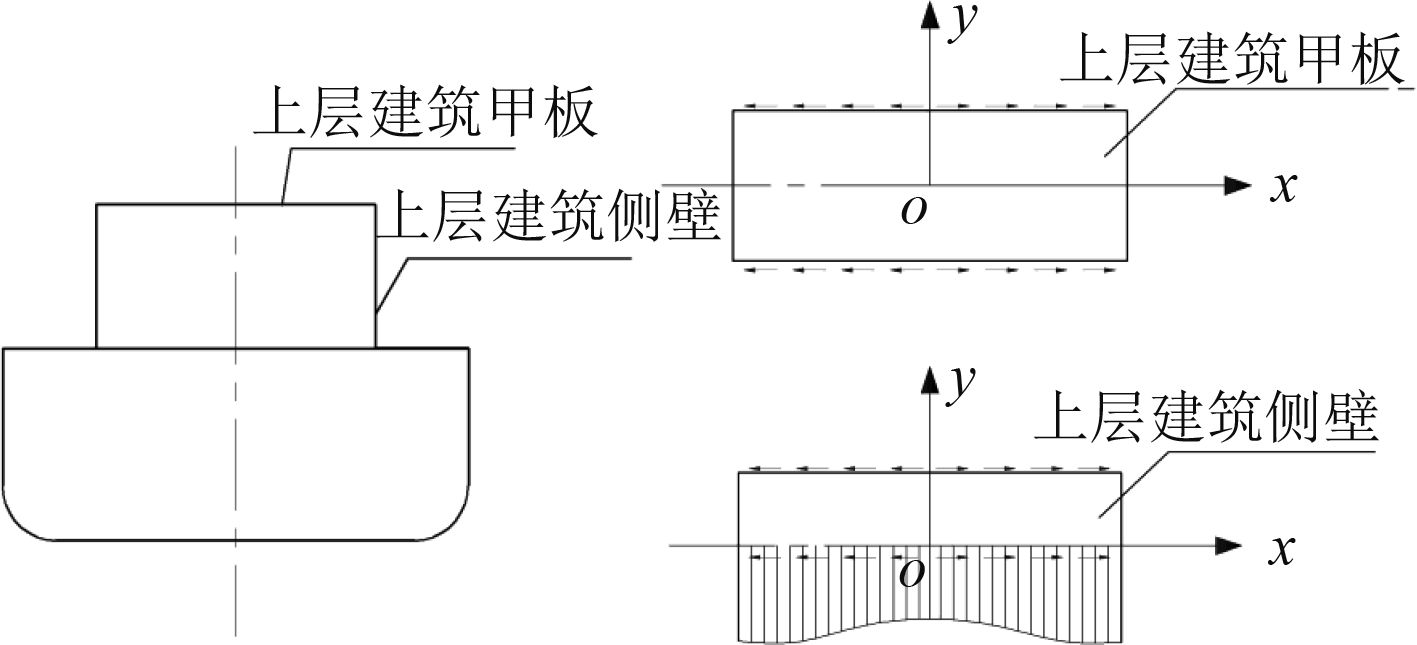图 1 计算上层建筑总强度的弹性力学模型 Fig. 1 Elasticity model for calculating the total strength of superstructure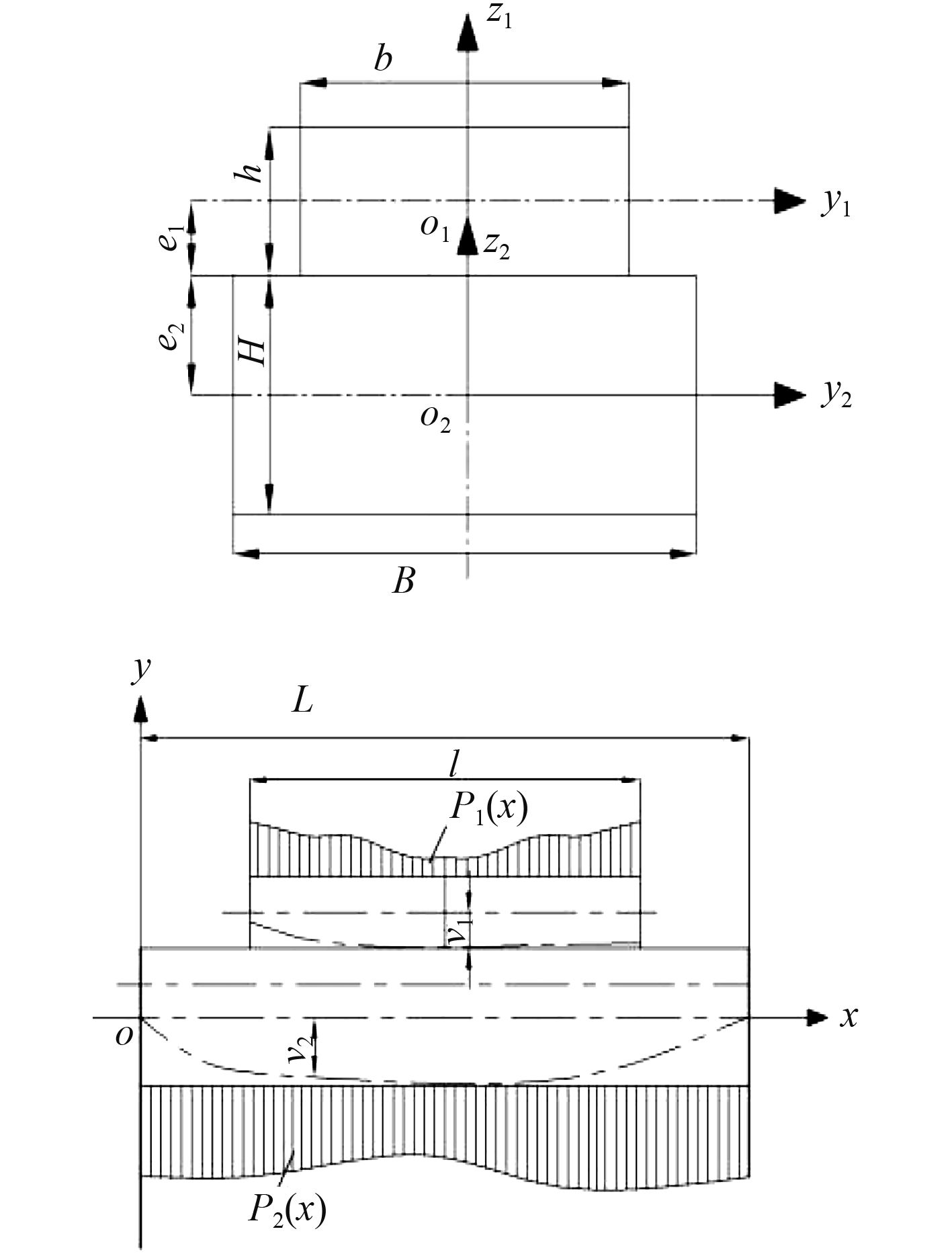图 2 计算上层建筑总强度的传统组合梁法力学模型 Fig. 2 The mechanical model of the traditional composite beam for calculating the total strength of the superstructure

 $\left\{ {\begin{array}{*{20}{c}} {{E_s}{i_s}{v_1}^{\prime \prime }（x） = \int_0^x {\int_0^x {（{p_1}（x） - p（x））{\rm{d}}x{\rm{d}}x + {e_1}\int_0^x {q（x）{\rm{d}}x} } } } \text{，}\\ {{E_0}{I_0}{v_2}^{\prime \prime }（x） = \int_0^x {\int_0^x {（{p_2}（x） - p（x））{\rm{d}}x{\rm{d}}x + {e_2}\int_0^x {q（x）{\rm{d}}x} } } } \text{。} \end{array}} \right.$ (1)

Crawford提出了改进的“双梁理论”，即假定上层建筑和主船体都是单独的梁，各自承受垂向力和水平力，主船体梁还承受外力矩，从而可以求得双梁的正应力、挠度和曲率。相比于传统的组合梁法，双梁理论并未采用弹性基础梁的微分方程，而是对垂向拉压力与水平剪力作Fourier变换，然后在若干假定的基础上建立平衡方程求解，因此该方法更加简单。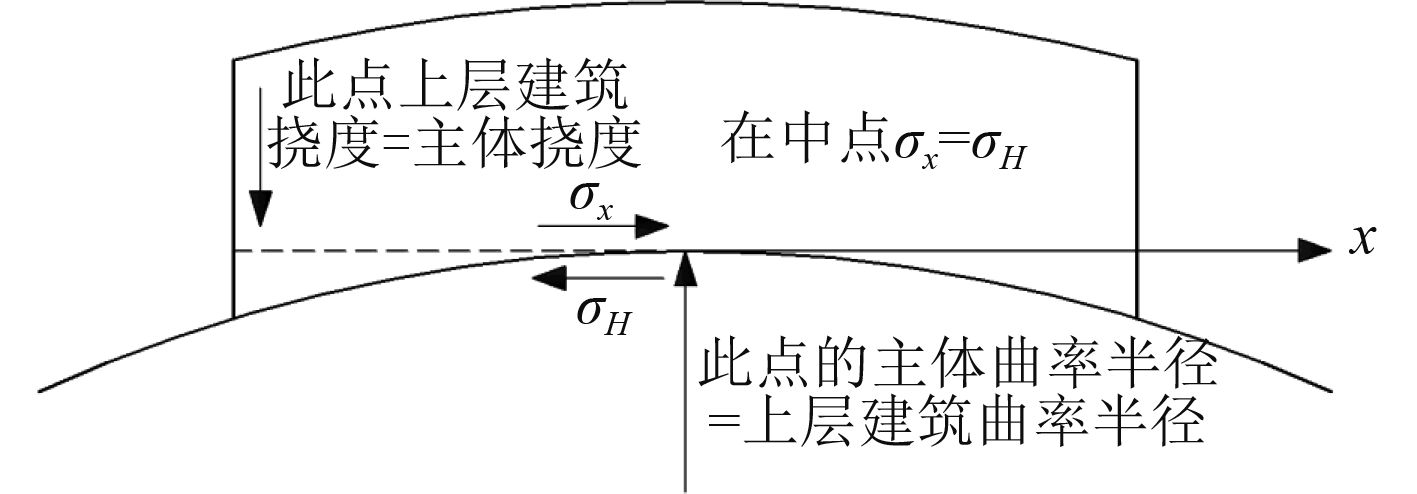图 3 计算上层建筑总强度的双梁理论模型 Fig. 3 Two-beam theoretical model for calculating the total strength of superstructure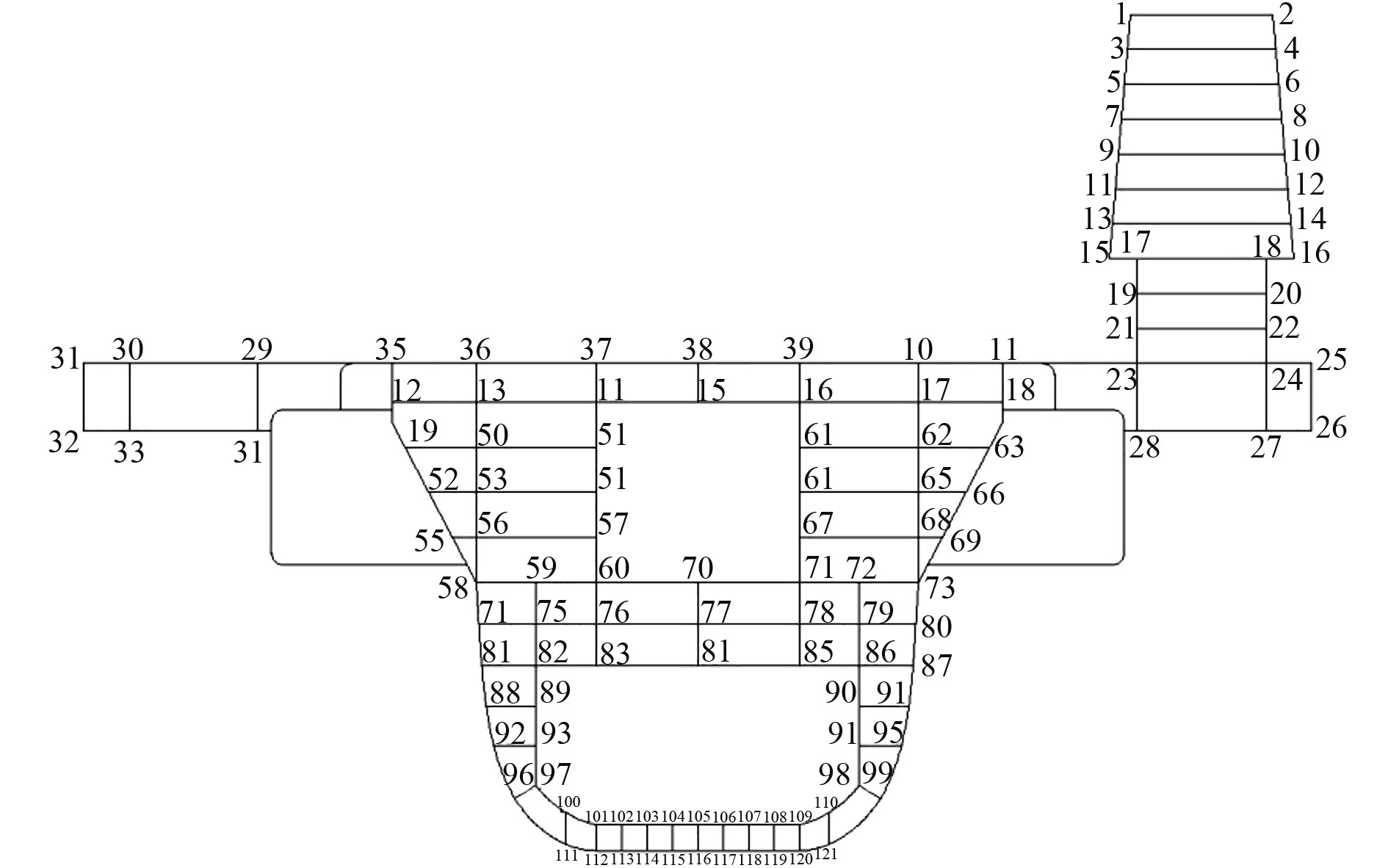图 4 主船体和上层建筑横剖面节点示意面 Fig. 4 Main hull and superstructure cross section node
 $\begin{gathered} f = \left\{ \omega \right\} = \sum\limits_{m = 1}^r {\left[ {\left[ {{C_1}} \right]\left[ {{C_2}} \right] \ldots } \right]} {\left\{ {\begin{array}{*{20}{c}} {\left\{ {{\delta _1}} \right\}} \\ {\left\{ {{\delta _2}} \right\}} \\ \vdots \end{array}} \right\}_m} \text{，}\hfill \\ {Y_m} = \sum\limits_{m = 1}^r {{Y_m}}\text{，} \sum\limits_{k = 1}^s {\left[ {{C_k}} \right]{{\left\{ {{\delta _k}} \right\}}_m}}\text{。} \hfill \\ \end{gathered}$ (2)

2 基于有限元理论的岛式上层建筑总强度计算

2.1 力学模型及基本理论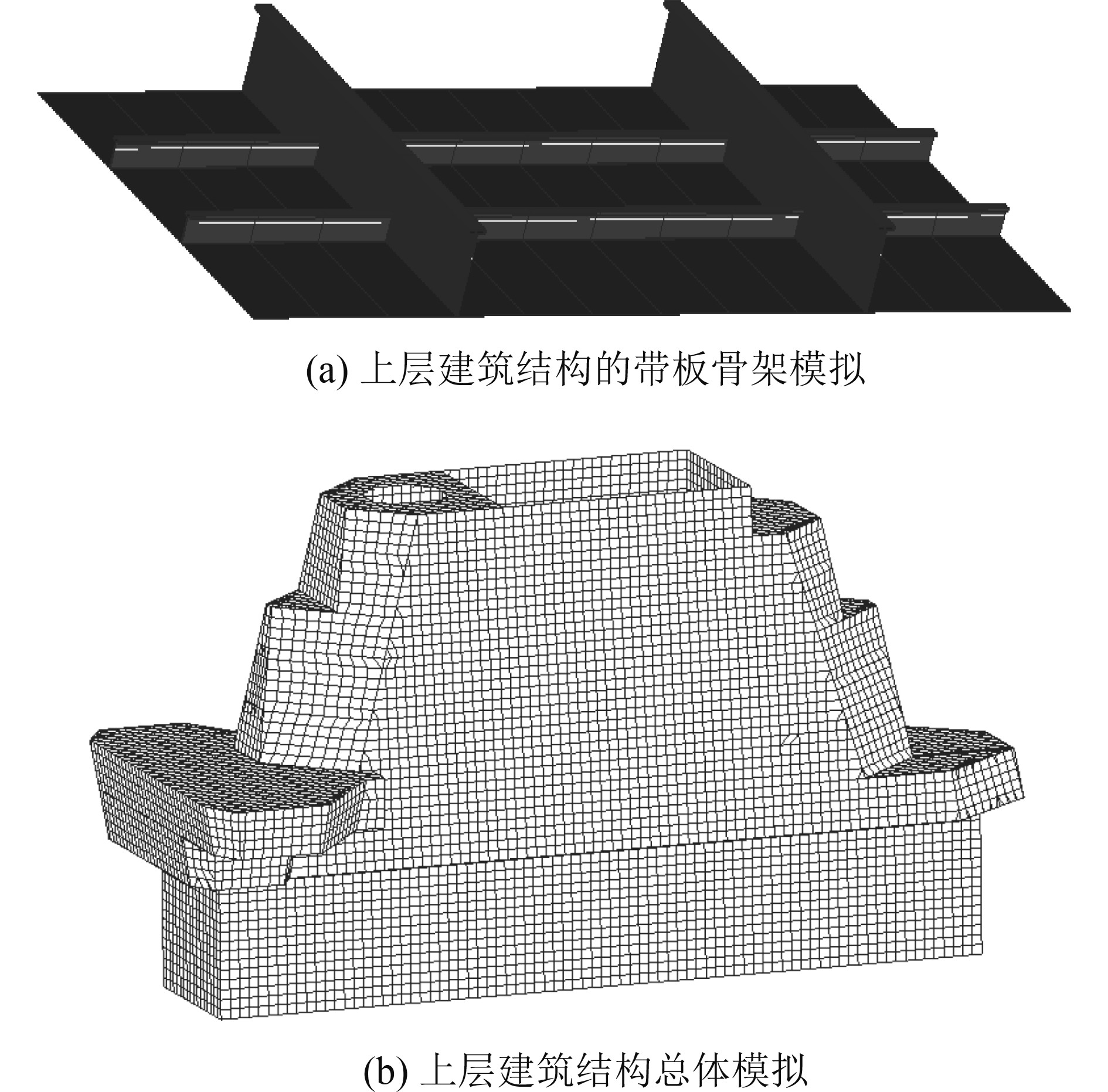图 5 计算上层建筑总强度的有限元模型 Fig. 5 Finite element model for calculating the total strength of superstructures
2.2 总强度载荷及加载方法

 $V = \frac{{10\;000}}{E}\int_0^x {\int_0^x {\frac{{{M_y}（x）}}{{{I_{Z0}}（x）}}{\rm{d}}{x^2} - } } \frac{{10\;000x}}{{EL}}\int_0^L {\int_0^x {\frac{{{M_y}（x）}}{{{I_{Z0}}（x）}}} } {\rm{d}}{x^2} \text{，}$ (4)
 ${M_y}（x） = {M_s}（x） + {M_{w2}}(x) \text{。}$ (5)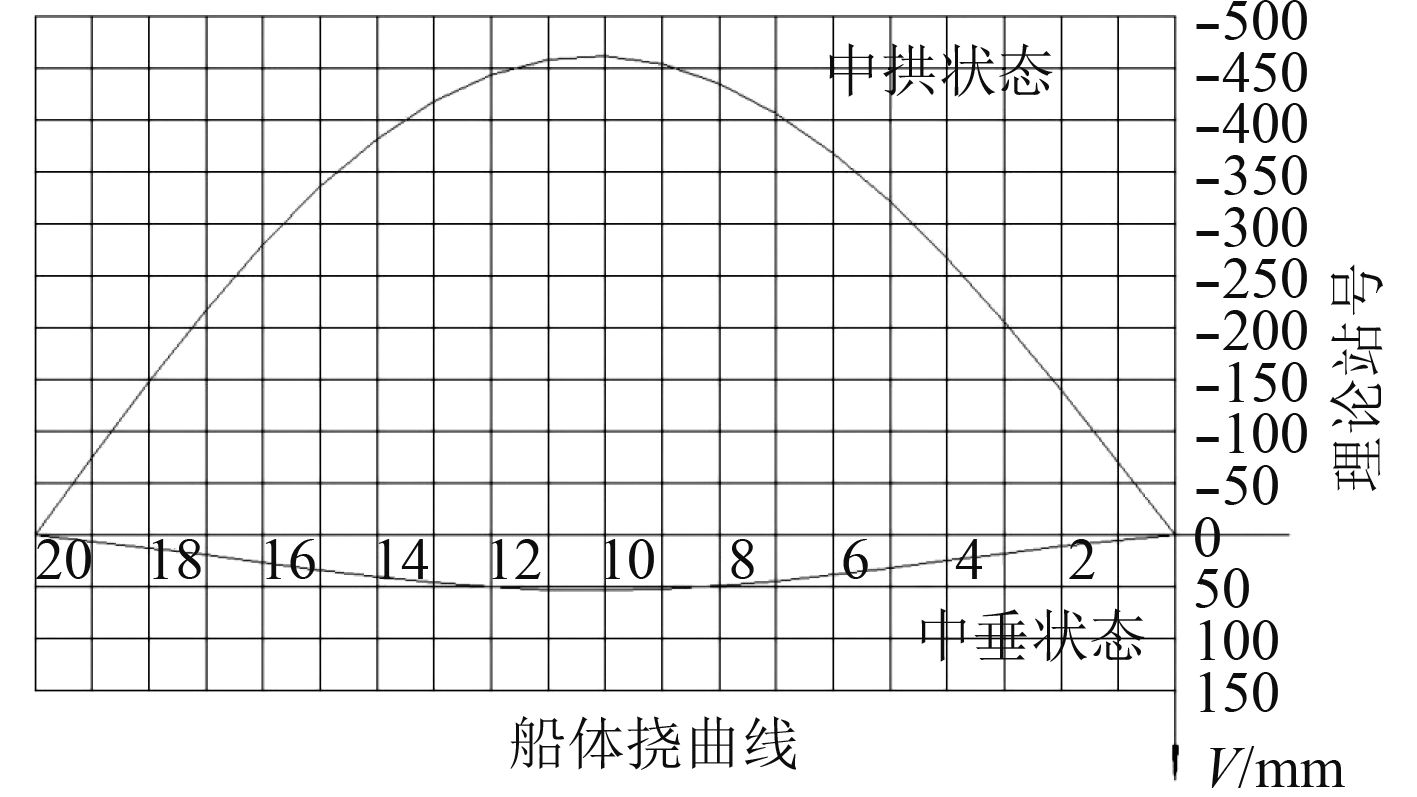图 6 中垂和中拱状态下的船体挠曲线 Fig. 6 In the middle and the arch state of the hull deflection curve表 1 中拱状态下上层建筑各站处的挠度值 Tab.1 The deflection value of each building in the superstructure in the arch state
2.3 计算结果及分析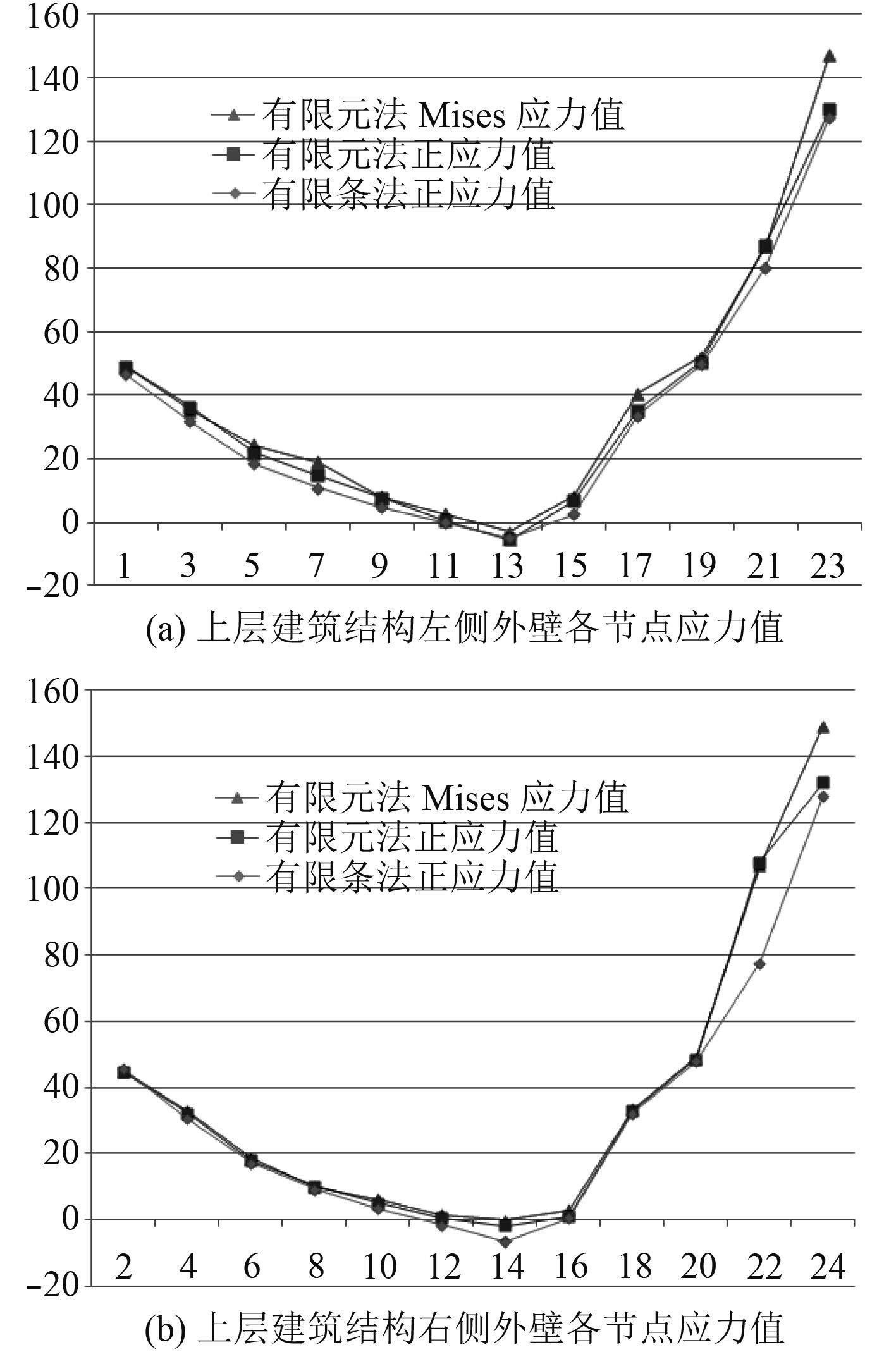图 7 上层建筑结构应力计算结果[注] Fig. 7 Calculation results of stress in superstructure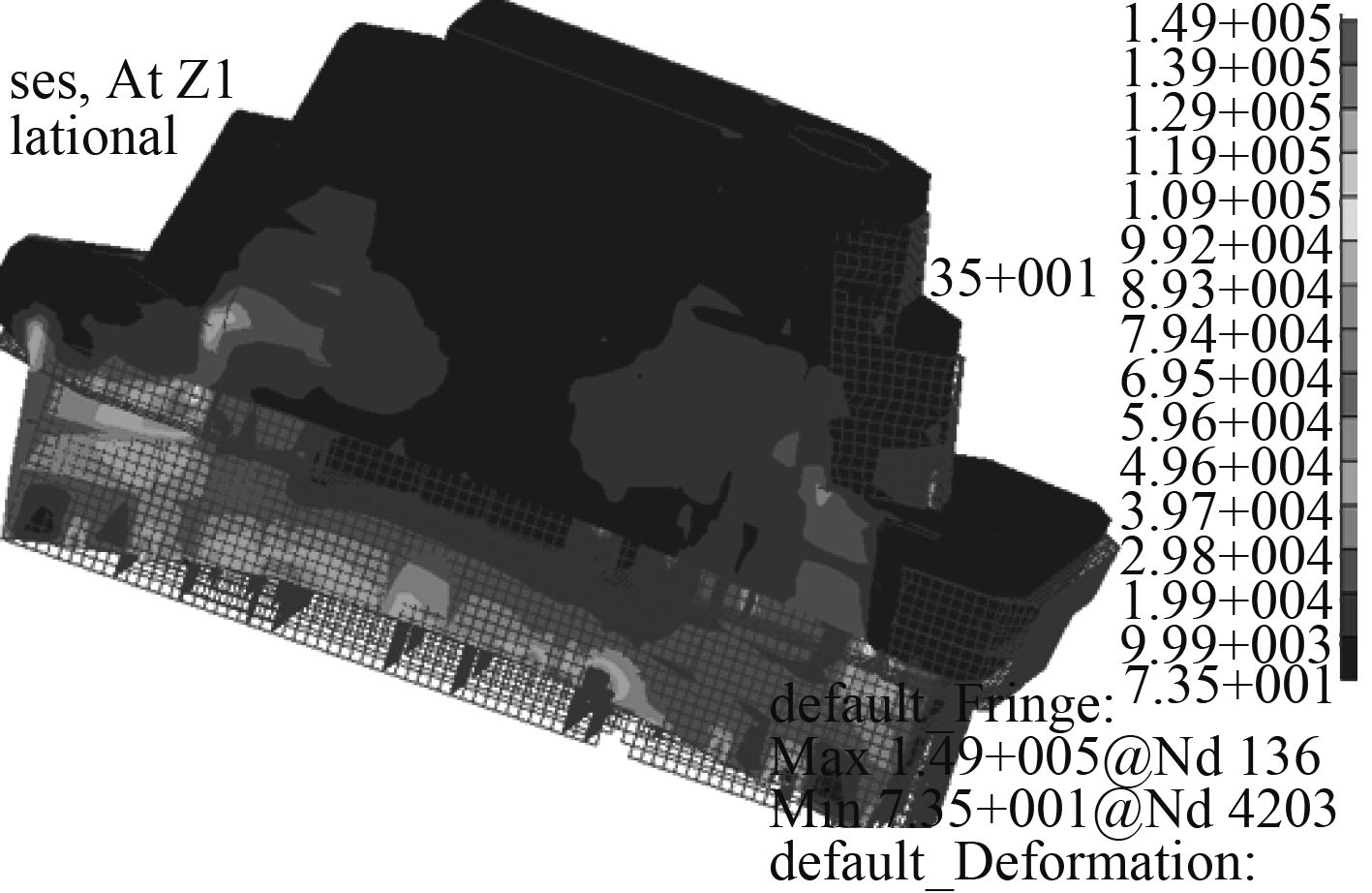图 8 上层建筑总强度有限元法计算结果 Fig. 8 Finite element method for calculating the total strength of superstructure

3 结　语

1）采用有限条法进行计算时，输入和输出数据少、矩阵阶数少，在计算的简便上有较大的优越性。而有限元法计算时，需要首先建立上层建筑的三维有限元模型，过程复杂，但是考虑到设计书中的校验顺序，在前期进行的船舶总强度计算中，已经建立起了整船有限元模型，可以十分方便地从中截取上层建筑部分，因此采用有限元法计算岛式上层建筑的强度问题也有很好的实用性。

2）将本文的计算结果与有限条法的计算结果进行对比可以发现，有限条法计算出的应力值偏小，注意到有限条法对实际结构有较大的简化，可以断定误差较大；有限元法填补了有限条法中只能纵向分条无法横向离散的缺点，计算出的应力值更加真实。

3）有限条法是对整体结构的一种平均计算，无法细化到某个或者某些结构，而有限元法则弥补了这点不足，不仅能计算总合应力和局部结构的应力，还能计算结构中的集中应力，为结构强度预报提供依据。

  海军装备部规范所. GJB.航母船体结构设计计算方法[S]. 北京: 总装备部军标出版社.  杨平, 吴凯. 上层建筑对船体总纵弯曲的效应分析[J]. 武汉交通科技大学学报, 1999(2): 31-36. YANG Ping, WU Kai. Analysis on the effect of superstructure on the longitudinal bending of hull[J]. Journal of Wuhan University of Communications Science and Technology, 1999(2): 31-36.  郑杰, 谢伟, 骆伟, 等. 穿浪双体船横向强度与扭转强度的有限元计算[J]. 中国舰船研究, 2010(5): 14-18. ZHENG Jie, XIE Wei, LUO Wei, et al. Finite element calculation of transverse strength and torsional strength of wave–piercing[J]. Chinese Journal of Ship Research, 2010(5): 14-18.  孙聪.内河船舶上层建筑对总纵强度的影响研究[D]. 哈尔滨: 哈尔滨工程大学, 2013.  王西典.计入有效度的强力上层建筑设计研究[D]. 上海: 上海交通大学, 2015.  CRAWFORD L. Theory of long ship’s superstructures[J]. SNAME, 1950(4): 693.  CHEUNG Y.K. 结构分析的有限条法[M]. 北京: 人民交通出版社, 1985.  陈庆强, 朱胜昌. 上层建筑一体化船型的船体梁总纵强度计算方法研究[J]. 船舶力学, 2011(10): 57-61. CHEN Qing-qiang, ZHU Sheng-chang. Study on calculating method of total longitudinal strength of hull girder with integrated building type[J]. Journal of Ship Mechanics, 2011(10): 57-61.  陆鑫森. 船舶上层建筑的强度问题[J]. 交通大学学报, 1953(3): 184-215. LU Xin-sen. Strength of ship superstructure[J]. Journal of Traffic University, 1953(3): 184-215.  陈庆强, 朱胜昌, 郭列, 等. 用整船有限元模型分析方法计算舰船的总纵强度[J]. 船舶力学, 2004(2): 79-85. CHEN Qing-qiang, ZHU Sheng-chang, GUO Lie, et al. Calculation of the total longitudinal strength of the ship by finite element model analysis method[J]. Journal of Ship Mechanics, 2004(2): 79-85. DOI:10.3969/j.issn.1007-7294.2004.02.011